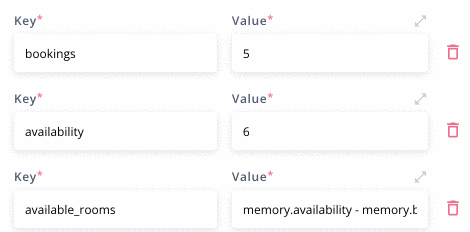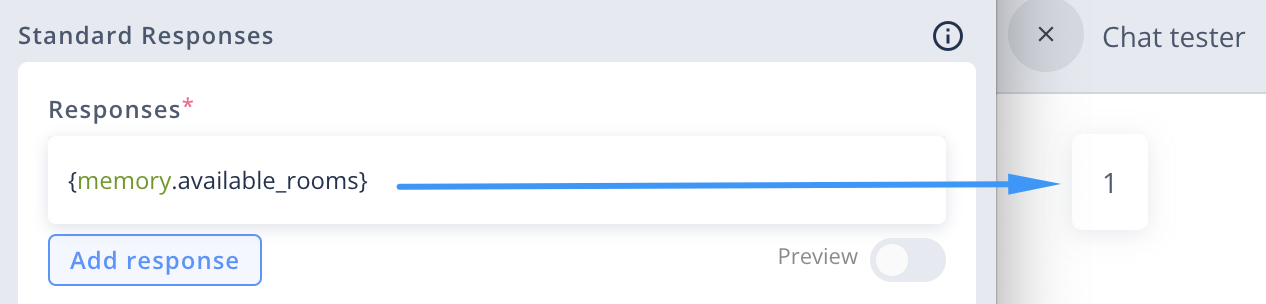# Operators

Operators are symbols (or keywords) that indicate various kinds of operations. Operators can be used in AEL expressions, both in Responses and input fields (eg. Memory).

The following list contains three types of operators:

• Arithmetic operators perform mathematical operations on numbers. Their results are numbers.
• Comparison operators compare values with each other. Their results are booleans (`true` or `false`).
• Logical operators connect expressions which results are booleans. Their results are also booleans (`true` or `false`).

# List of operators

## Arithmetic operators

Arithmetic operators perform mathematical operations on numbers. These numbers are called operands. Operands can be literal numbers (eg. `5`, `0`, `1.5`, `319`) or numbers stored as variables (eg. `memory.someSavedNumber`, `nlu.variables.numeral.value`).

The following table contains all of the arithmetic operators:

OperatorDescription
+Addition. For example, `5 + 4` will return `9`
-Subtraction. For example, `5 - 4` will return `1`
*Multiplication. For example, `5 * 4` will return `20`
/Division. For example, `5 / 4` will return `1.25`
%Modulus (remainder). For example, `5 % 4` will return `1`

Sequence of operations

You can perform multiple mathematical operations at once. Normal rules of sequence of operations apply. You can use brackets `()` to change the sequence of operations, eg. `(memory.numberOfCases + 1) * 5`.

### Examples

``````1 + 5 * 2
//Result:
11

(1 + 5) * 2
//Result
12
``````
``````//For:
//memory.numberOfCases = 3
//nlu.variables.numeral.value = 15:

(memory.numberOfCases + 1) * nlu.variables.numeral.value
//Result:
60

``````

## Comparison operators

Comparison operators compare two values with each other. Their result is always a bollean (`true` or `false`), based on if the comparison is true.

For example, `1 = 3` will return `false`, and `3 > 1` will return `true`.

The following table contains all of the comparison operators:

OperatorDescription
=Equality operator. Compares the equality of two operands of the same type. Returns `true` if they are identical, and `false` if not. You can compare the two values of each of the following data types: numbers, strings, dates, arrays and objects.
!=Inequality operator. Compares inequality of two operands. It will return `false` where `=` would return `true`. You can compare the two values of each of the following data types: numbers, strings, dates, arrays and objects.
>Greater than. Returns a boolean value `true` if the left-side value is greater than the right-side value; otherwise, returns `false`. You can compare the two values of each of the following data types: numbers, dates.
<Less than.Returns a boolean value `true` if the left-side value is less than the right-side value; otherwise, returns `false`. You can compare the two values of each of the following data types: numbers, dates.
>=Greater than or equal. Returns a boolean value `true` if the left-side value is greater than or equal to the right-side value; otherwise, returns `false`. You can compare the two values of each of the following data types: numbers, dates.
<=Less than or equal. Returns a boolean value `true` if the left-side value is less than or equal to the right-side value; otherwise, returns `false`. You can compare the two values of each of the following data types: numbers, dates.
``````1 = 2
//Result:
false

22.4 > 4
//Result:
true

5 <= 700
true
``````
``````"Pam Beasley" = "Pam Halpert"
//Result:
false

"Oscar Martinez" = "Oscar Martinez"
//Result:
true
``````
``````system.currentTime > "1992-10-10".toDateTime()
//Result:
true

system.currentTime.plusDays(3) < system.currentTime
//Result:
false

system.currentTime.plusMinutes(60) > system.currentTime
//Result:
true
``````
``````[1,2,3] = [1,2,3]
//Result:
true

{"name": "Jim", "surname": "Halpert"} = {"name": "Jim"}
//Result:
false

{"n": 1} = {"n": 1}
//Result:
true
``````

### Comparing values stored as variables

You can compare literal values (eg. `1 > 2`) as well as values stored as variables (eg. `system.userInput = 'hello'`).

``````//for:
//memory.numberOfCases = 3
//nlu.variables.numeral.value = 2

memory.numberOfCases >= nlu.variables.numeral.value
//Result:
true
``````
``````//for:
//system.userInput = "Michael"
//memory.name = "Michael"
//memory.surname = "Scott"

system.userInput = memory.surname
//Result:
false

system.userInput = memory.name
//Result:
true
``````

## Logical operators

Logical operators `and` and `or` are used to connect two or more expressions.

The result of each of the connected expressions has to be a boolean (`true` or `false`). If any of these expressions does not result in a boolean, such error will appear: `Logical operations are only supported by boolean values`.

The result of using logical operator is always a boolean.

OperatorDescription
andIt results in `true` if both of the expressions result in `true`. Otherwise, it results in `false`.
orIt results in `true` if at least one of the expressions results in `true`. Otherwise, it results in `false`.
notChanges `true` to `false` and vice versa, eg. `not (5 > 1)` will result in `false`, and `not false` will result in `true`.
``````true and false
//Result:
false

true and 1 > 2
//Result:
true

system.userInput = "Michael" and memory.paperAmount = 30
//Result if both are true:
true
//Result if one of them is false:
false
//Result if both are false:
false
``````
``````true or false
//Result:
true

true or 1 > 2
//Result:
true

system.userInput = "Michael" or memory.paperAmount = 30
//Result if both are true:
true
//Result if one of them is false:
true
//Result if both are false:
false
``````
``````not (false)
//Result:
true

not (2 > 1)
//Result:
false

//for: system.userInput = "Dwight"
not (system.userInput = "Dwight")
//Result:
false
``````

### Resolving multiple logical operators

When you use multiple logical operators in one expression, they are resolved according to short-circuit evaluation. This means that they are resolved one by one, starting from the first one on the left.

The `and` operator has a higher precedence than the `or` operator, meaning the `and` operator is executed before the `or` operator.

If the end result is known before resolving all of the expressions connected by `and` or `or`, the rest of these expressions won't be resolved at all. Thanks to that, you don't need to worry about them causing errors.

``````true and true and false
//Result:
false

true or false or false
//Result:
true

false and true or false
//Result:
false
``````
``````nlu.variables.numeral.value > 2 and system.userInput = "Scott" or system.userInput = "Michael"
//Result if all are true:
true
``````
``````//for:
//memory.numbers = [23, 45]

memory.numbers = 9
//Such expression will throw an error as there is no element of index 2 in the array memory.numbers.
//To avoid this error, you can add another condition before which will check
//if the array has an element of index 2:
memory.numbers.length >= 3 and memory.numbers = 9
//Result:
false
``````

You can use brackets `()` to change the order of evaluation of logical operators.

``````true or false and false or false
//Result:
true

//But:
(true or false) and (false or false)
//Result:
false

``````

# Using operators

All operators can be used in any AEL expression. They're mainly used in Responses and Remory input fields. Besides that, logical and comparison operators are also used in dropdowns lists of ondition fields.

## Using operators in Responses

In the example below, an expression `1 = 2` contains comparison operator of equality `=`. The expression has been added to the response input by using curly brackets `{}`. The bot's response will be `"false"` as `2` is not equal to `1`.

In the next example, an expression `if (system.currentTime.getDayOfWeek() = 7) "Sunday" else "not Sunday"` uses comparison operator of equality `=` to set a condition for if statement. The expression has beed added to the Response by using curly brackets `{}`. The bot's response will be "Today is Sunday." on the seventh day of the week and "Today is not Sunday." on any other day.

In the example below, the subtraction operator `-` is used to define memory value `memory.available_rooms` by substracting the number of bookings received from the number of total available rooms.And then, adding the expression `{memory.available_rooms}` in the response, where `available_rooms` is the key name of the result value of the subtraction, prints the result `1` as the bot's response.## Using operators in Memory values

In the example below, a result of `memory.userInputIsEmpty` will be `true` if the user's input is empty, and `false` if it's not. In Memory value inputs, we don't need to add curly brackets `{}` to use AEL.